Home
Hostname: page-component-78bd46657c-lnsrr Total loading time: 1.051 Render date: 2021-05-08T05:21:28.097Z Has data issue: true Feature Flags: { "shouldUseShareProductTool": true, "shouldUseHypothesis": true, "isUnsiloEnabled": true, "metricsAbstractViews": false, "figures": false, "newCiteModal": false, "newCitedByModal": true }Compositio Mathematica

# On lower bounds for the Ihara constants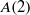$A(2)$ and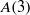$A(3)$

Published online by Cambridge University Press:  03 May 2013

Corresponding

## Abstract

Let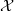$\mathcal{X}$ be a curve over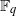${ \mathbb{F} }_{q}$ and let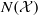$N( \mathcal{X} )$,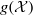$g( \mathcal{X} )$ be its number of rational points and genus respectively. The Ihara constant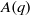$A(q)$ is defined by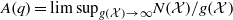$A(q)= {\mathrm{lim~sup} }_{g( \mathcal{X} )\rightarrow \infty } N( \mathcal{X} )/ g( \mathcal{X} )$. In this paper, we employ a variant of Serre’s class field tower method to obtain an improvement of the best known lower bounds on$A(2)$ and$A(3)$.

## MSC classification

Type
Research Article
Information
Compositio Mathematica , July 2013 , pp. 1108 - 1128

## References

Angles, B. and Maire, C., A note on tamely ramified towers of global function fields, Finite Fields Appl. 8 (2002), 207215.CrossRefGoogle Scholar
Auer, R., Ray class fields of global function fields with many rational places, Acta Arith. 95 (2000), 97122.Google Scholar
Cassels, J. W. S. and Fröhlich, A., Algebraic number theory (London Mathematical Society, Providence, RI, 2010).Google Scholar
Drinfel’d, V. G. and Vlăduţ, S. G., The number of points of an algebraic curve, Funktsional. Anal. i Prilozhen. 17 (1983), 6869.Google Scholar
Garcia, A., Stichtenoth, H., Bassa, A. and Beelen, P., Towers of function fields over non-prime finite fields, Preprint, math.AG/1202.5922.Google Scholar
Gaschütz, W. and Newman, M. F., On presentations of finite$p$-groups, J. Reine Angew. Math. 245 (1970), 172176.Google Scholar
Golod, E. S. and Safarevich, I. R., On the class field tower, Izv. Akad. Nauk SSSR Ser. Mat. 28 (1964), 261272.Google Scholar
Hajir, F. and Maire, C., Asymptotically good towers of global fields, in European congress of mathematics, Barcelona, 2000, vol. II, Progress in Mathematics, vol. 202 (Birkhäuser, Basel, 2001), 207218.Google Scholar
Hajir, F. and Maire, C., Extensions of number fields with wild ramification of bounded depth, Int. Math. Res. Not. IMRN 2002 (2002), 667696.CrossRefGoogle Scholar
Hartshorne, R., Algebraic geometry, Graduate Texts in Mathematics, vol. 52 (Springer, New York, 1977).CrossRefGoogle Scholar
Hoechsmann, K., Zum Einbettungsproblem, J. Reine Angew. Math. 229 (1968), 81106.Google Scholar
Ihara, Y., Some remarks on the number of rational points of algebraic curves over finite fields, J. Fac. Sci. Univ. Tokyo Sect. IA 28 (1981), 721724.Google Scholar
Kuhnt, T., Generalizations of Golod–Shavarevich and applications, PhD thesis, University of Illinois at Urbana-Champaign (2002).Google Scholar
Lang, S., Algebra, Graduate Texts in Mathematics, vol. 211, third edition (Springer, New York, 2002).CrossRefGoogle Scholar
Li, W.-C. W., Upper and lower bounds for$A(q)$, in Recent trends in coding theory and its applications, AMS/IP Studies in Advanced Mathematics, vol. 41 (American Mathematical Society, Providence, RI, 2007), 1523.CrossRefGoogle Scholar
Li, W.-C. W. and Maharaj, H., Coverings of curves with asymptotically many rational points, J. Number Theory 96 (2002), 232256.CrossRefGoogle Scholar
Neukirch, J., Über das Einbettungsproblem der algebraischen Zahlentheorie, Invent. Math. 21 (1973), 59116.CrossRefGoogle Scholar
Neukirch, J., Schmidt, A. and Wingberg, K., Cohomology of number fields, Grundlehren der Mathematischen Wissenschaften [Fundamental Principles of Mathematical Sciences], vol. 323, second edition (Springer, Berlin, 2008).CrossRefGoogle Scholar
Niederreiter, H. and Xing, C., Towers of global function fields with asymptotically many rational places and an improvement on the Gilbert–Varshamov bound, Math. Nachr. 195 (1998), 171186.CrossRefGoogle Scholar
Niederreiter, H. and Xing, C., Curve sequences with asymptotically many rational points, in Applications of curves over finite fields, Seattle, WA, 1997, Contemporary Mathematics, vol. 245 (American Mathematical Society, Providence, RI, 1999), 314.CrossRefGoogle Scholar
Niederreiter, H. and Xing, C., Rational points on curves over finite fields: theory and applications, London Mathematical Society Lecture Note Series, vol. 285 (Cambridge University Press, Cambridge, 2001).CrossRefGoogle Scholar
Schoof, R., Algebraic curves over${\mathbf{F} }_{2}$ with many rational points, J. Number Theory 41 (1992), 614.CrossRefGoogle Scholar
Serre, J.-P., Local fields, Graduate Texts in Mathematics, vol. 67 (Springer, New York, 1979).CrossRefGoogle Scholar
Serre, J.-P., Rational points on curves over finite fields, lecture notes, Harvard University (1985).Google Scholar
Serre, J.-P., Galois cohomology, Springer Monographs in Mathematics, English edition (Springer, Berlin, 2002).Google Scholar
Stichtenoth, H., Algebraic function fields and codes, Graduate Texts in Mathematics, vol. 254, second edition (Springer, Berlin, 2009).Google Scholar
Temkine, A., Hilbert class field towers of function fields over finite fields and lower bounds for$A(q)$, J. Number Theory 87 (2001), 189210.CrossRefGoogle Scholar
Tsfasman, M. A., Vlăduţ, S. G. and Zink, Th., Modular curves, Shimura curves, and Goppa codes, better than Varshamov–Gilbert bound, Math. Nachr. 109 (1982), 2128.CrossRefGoogle Scholar
Weil, A., Sur les courbes algébriques et les variétés qui s’en déduisent, Actualités Sci. Ind., no. 1041; Publ. Inst. Math. Univ. Strasbourg, vol. 7 (1945) (Hermann, Paris, 1948).Google Scholar
Weibel, C. A., An introduction to homological algebra, Cambridge Studies in Advanced Mathematics, vol. 38 (Cambridge University Press, Cambridge, 1994).CrossRefGoogle Scholar
Xing, C. and Yeo, S. L., Algebraic curves with many points over the binary field, J. Algebra 311 (2007), 775780.CrossRefGoogle ScholarYou have Access

# Send article to Kindle

Note you can select to send to either the @free.kindle.com or @kindle.com variations. ‘@free.kindle.com’ emails are free but can only be sent to your device when it is connected to wi-fi. ‘@kindle.com’ emails can be delivered even when you are not connected to wi-fi, but note that service fees apply.

Find out more about the Kindle Personal Document Service.

On lower bounds for the Ihara constants$A(2)$and$A(3)$
Available formats
×

# Send article to Dropbox

To send this article to your Dropbox account, please select one or more formats and confirm that you agree to abide by our usage policies. If this is the first time you use this feature, you will be asked to authorise Cambridge Core to connect with your <service> account. Find out more about sending content to Dropbox.

On lower bounds for the Ihara constants$A(2)$and$A(3)$
Available formats
×

# Send article to Google Drive

To send this article to your Google Drive account, please select one or more formats and confirm that you agree to abide by our usage policies. If this is the first time you use this feature, you will be asked to authorise Cambridge Core to connect with your <service> account. Find out more about sending content to Google Drive.

On lower bounds for the Ihara constants$A(2)$and$A(3)$
Available formats
×
×